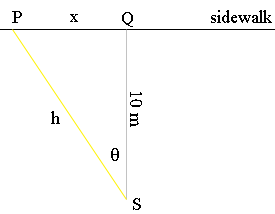SEARCH HOMEMath Central Quandaries & QueriesQuestion from Meredith, a student: A searchlight is position 10 meters from a sidewalk. A person is walking along the sidewalk at a constant speed of 2 meters per second. The searchlight rotates so that it shines on the person. Find the rate at which the searchlight rotates when the person is 25 meters from the searchlight.Hi Meredith,

Here is my diagram.$S$ is the searchlight $P$ is the person and the triangle $QPS$ is a right triangle. $\theta$ is measured counterclockwise and hence if the person is walking towards $Q$ then $\large {\frac{d\theta}{dt}}$ and $\large{ \frac{dx}{dt}}$ are both negative where $t$ is time in seconds. You want $\large \frac{d\theta}{dt}$ when $h$ is 25 meters. Since you know $\large \frac{dx}{dt}$ I would look for a relationship between $x$ and $\theta.$ What I see is that$\frac{x}{10} = \tan \theta$ so $x = 10 \tan \theta.$

Differentiate both sides of the above equation with respect to $t$ to obtain a relationship involving $\large \frac{dx}{dt}, \frac{d\theta}{dt},$ and $\theta.$ You will need to evaluate $\theta$ when $h$ is 25 meters.

PennyMath Central is supported by the University of Regina and The Pacific Institute for the Mathematical Sciences.